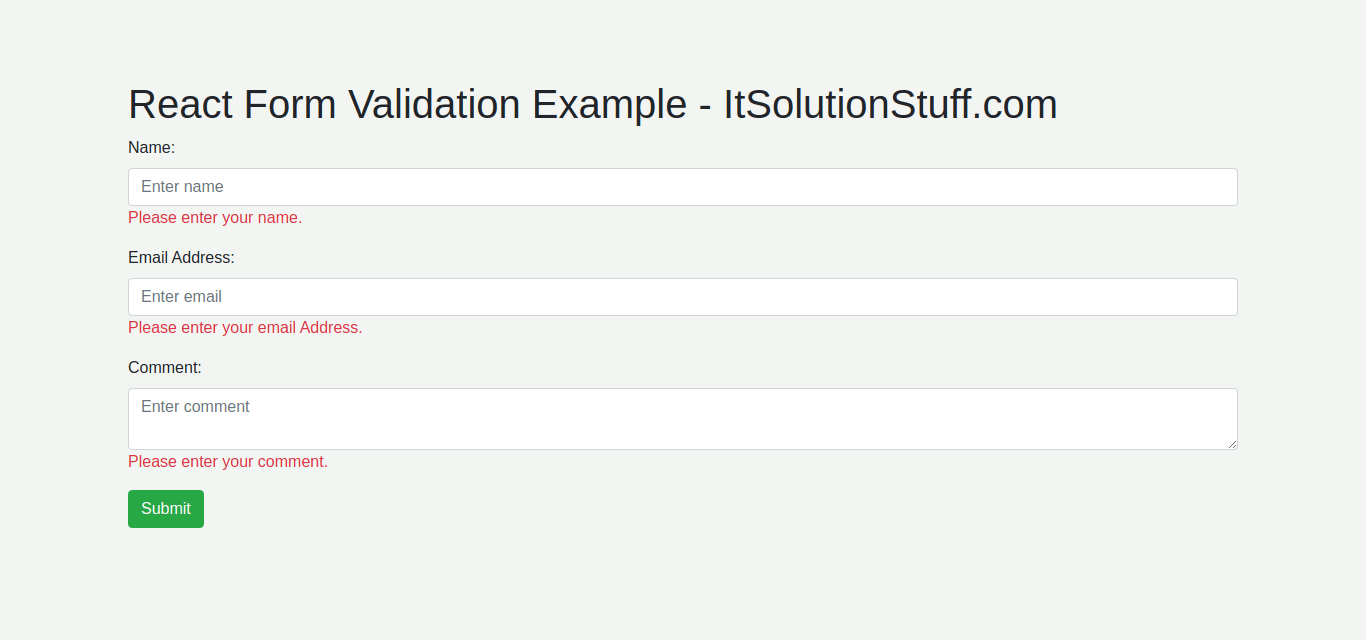# React Form Validation Tutorial Example

By Hardik Savani September 6, 2020 Category : React JS

Now, let's see tutorial of how to add form validation in react js. we will help you to give example of react form validation example. This article will give you simple example of react input validation example. if you want to see example of react bootstrap form validation example then you are a right place. Let's see bellow example react input field validation example.

Form validation is a primary part of any application. we always require to add input validation when we are adding form in our application. There is a some simple validation like require, email, phone number, only number validation is almost we need to add. When ever user enter wrong value on input field then we need to display error messages.

So, here i will give you step by step instruction of how to add bootstrap form validation in react js application. in this example we will take name, email and comment input and add validation for require and email now. also display error messages if they enter wrong values.

Let's follow bellow step and you will find preview as bellow:

Preview:Step 1: Install React App

In our first step, we need to download react js fresh app using bellow command, if you didn't install yet then.

`npx create-react-app my-app`

Step 2: Create DemoForm Component

In this step, we will create DemoForm.js component file and we will write code of form validation. so let's add code as bellow:

src/DemoForm.js

`import React from 'react';  class DemoForm extends React.Component {    constructor() {    super();    this.state = {      input: {},      errors: {}    };         this.handleChange = this.handleChange.bind(this);    this.handleSubmit = this.handleSubmit.bind(this);  }       handleChange(event) {    let input = this.state.input;    input[event.target.name] = event.target.value;      this.setState({      input    });  }      handleSubmit(event) {    event.preventDefault();      if(this.validate()){        console.log(this.state);          let input = {};        input["name"] = "";        input["email"] = "";        input["comment"] = "";        this.setState({input:input});          alert('Demo Form is submited');    }  }    validate(){      let input = this.state.input;      let errors = {};      let isValid = true;        if (!input["name"]) {        isValid = false;        errors["name"] = "Please enter your name.";      }        if (!input["email"]) {        isValid = false;        errors["email"] = "Please enter your email Address.";      }        if (typeof input["email"] !== "undefined") {                  var pattern = new RegExp(/^(("[\w-\s]+")|([\w-]+(?:\.[\w-]+)*)|("[\w-\s]+")([\w-]+(?:\.[\w-]+)*))(@((?:[\w-]+\.)*\w[\w-]{0,66})\.([a-z]{2,6}(?:\.[a-z]{2})?)\$)|(@\[?((25[0-5]\.|2[0-4][0-9]\.|1[0-9]{2}\.|[0-9]{1,2}\.))((25[0-5]|2[0-4][0-9]|1[0-9]{2}|[0-9]{1,2})\.){2}(25[0-5]|2[0-4][0-9]|1[0-9]{2}|[0-9]{1,2})\]?\$)/i);        if (!pattern.test(input["email"])) {          isValid = false;          errors["email"] = "Please enter valid email address.";        }      }        if (!input["comment"]) {        isValid = false;        errors["comment"] = "Please enter your comment.";      }        this.setState({        errors: errors      });        return isValid;  }       render() {    return (      <div>        <h1>React Form Validation Example - ItSolutionStuff.com</h1>        <form onSubmit={this.handleSubmit}>            <div class="form-group">            <label for="name">Name:</label>            <input               type="text"               name="name"               value={this.state.input.name}              onChange={this.handleChange}              class="form-control"               placeholder="Enter name"               id="name" />                <div className="text-danger">{this.state.errors.name}</div>          </div>            <div class="form-group">            <label for="email">Email Address:</label>            <input               type="text"               name="email"               value={this.state.input.email}              onChange={this.handleChange}              class="form-control"               placeholder="Enter email"               id="email" />                 <div className="text-danger">{this.state.errors.email}</div>          </div>            <div class="form-group">            <label for="comment">Comment:</label>            <textarea               name="comment"              value={this.state.input.comment}               onChange={this.handleChange}              placeholder="Enter comment"              class="form-control" />                <div className="text-danger">{this.state.errors.comment}</div>          </div>                       <input type="submit" value="Submit" class="btn btn-success" />        </form>      </div>    );  }}  export default DemoForm;`

Step 3: Import Component

In this step, we will import DemoFormcomponent in index.js main file. so let's update index.js file as bellow:

Here, i used bootstrap with react. so, if you want to use bootstrap in your app too then follow this tutorial: Install Bootstrap 4 in React JS.

src/index.js

`import React from 'react';import ReactDOM from 'react-dom';import * as serviceWorker from './serviceWorker';import 'bootstrap/dist/css/bootstrap.min.css';  import DemoForm from './DemoForm';  ReactDOM.render(  <React.StrictMode>    <div className="container">       <DemoForm />    </div>  </React.StrictMode>,  document.getElementById('root'));  serviceWorker.unregister();`

Now we are ready to run our application, so let's run using bellow command:

`npm start`

Open bellow url:

`http://localhost:3000`